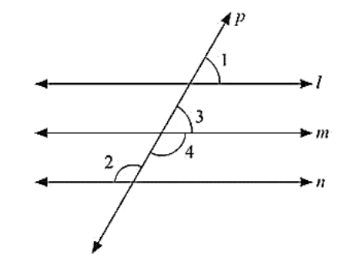# In the below fig, if l ∥ m ∥ n and ∠1 = 60°. Find ∠2.

Question:

In the below fig, if l ∥ m ∥ n and ∠1 = 60°. Find ∠2.Solution:

Since l parallel to m and p is the transversal

Therefore, Given: l ∥ m ∥ n

∠1 = 60°

To find ∠2

∠1 = ∠3 = 60°             [Corresponding angles]

Now, ∠3and∠4 are linear pair of angles

∠3 + ∠4 = 180°

60 + ∠4 = 180

∠4 = 180 - 60

⟹ 120

Also, m ∥ n and P is the transversal

Therefore ∠4 = ∠2 = 120 (Alternative interior angle]

Hence 2∠2 = 120